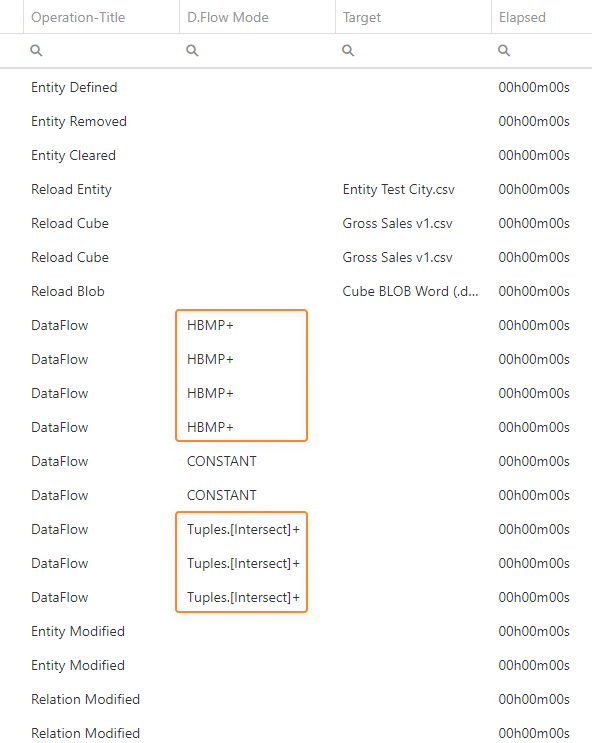# The Dataflow action

## Introduction

The Dataflow action is a very simple yet powerful feature of Board, a step that allows organizations to implement sophisticated business models without having to use complex programming languages.
The main function of the Dataflow is to calculate data of a Cube based on data of other Cubes and a formula. For example, sales margin values could be calculated in a Margin Cube by taking values in the Gross Sales Cube and subtracting the values in the Cost of Goods sold Cube (Margin Cube = Gross Sales Cube - Cost of Goods sold Cube).

The Dataflow action can be used to copy a slice of one Cube to another Cube, calculate output Cubes, process text and date Cubes, and much more.

## How the Dataflow works

The most basic Dataflow configuration requires a Layout definition and an expression (also called "formula"). Various optional settings are available to extend or limit the cells processed in the calculation or to shift the results of the calculation into the target Cube along its time dimension. See the following paragraphs for more details.

The expression syntax mirrors the algorithm syntax used in the Layout. You can also use substitution formulas and Metadata variables in the "Expression" field.
The functions listed in this document are also supported in the expression: these functions will only work with cells as input (not cell ranges, text strings , or others).

When the Dataflow is triggered in a Procedure, the following operations are performed:

1. The target Cube is cleared based on the currently active Select, unless the target Cube is also referenced in the formula (i.e. it is a "factor Cube")
2. The values in the factor Cubes are processed for all Entities in the structure of the target Cube, at the highest level of granularity. This is similar to what would happen if you added the factor Cubes to a Layout as Blocks and set all the Entities in the target Cube structure in "By Row" in the Axis area.
The result is a set of combinations of the members of Entities that are in the Structure of the target Cube which have a non-zero value on at least one of the Blocks of the Layout and are in the currently active Select.
Each of those combinations is called a tuple and the entire set of resulting tuples is the calculation domain (or, in other words, the set of all possible inputs for the formula)
3. The formula is executed on each tuple in the calculation domain and the results are written in the target Cube.

The Dataflow step may use different types of algorithms for its calculations depending on certain conditions related to the structures of the Cubes involved and the formula. The process described above is the most common one and uses the algorithm called "Tuples [union]".
You can view the algorithm type used by the step in the Logs section of the Data model. See the "Types of algorithms" paragraph in this page for more details.
Cubes whose Structure cannot be made congruent with the target Cube by aggregation do not contribute to the generation of the set of tuples, unless their missing Entities are among those set in the extend option.
The calculation domain can be limited or expanded through the options available under the "CALCULATION DOMAIN" menu.
If there is at least one Entity Block in the Dataflow Layout, all Entities will be automatically set in the extend option.

If the Dataflow is configured in a Capsule Procedure, a Data model drop-down menu on the upper right corner allows you to select the Data model on which it will be executed. The selected Data model is also automatically set in the Layout Editor: if you select another Data model in the drop-down menu, existing Layout definitions are cleared.

Example

A simple example of the capabilities of the Dataflow is the following.

In a scenario where you have the Cube "Quantity" (dimensioned by Month, Product, and Customer), the Cube "Product Standard cost" (dimensioned by Year and Product), and the Cube "Cost of Goods Sold" (dimensioned by Month, Product, Customer), you can calculate the values that will be stored in the Cube "Cost of Goods Sold" by multiplying the values in the Cube "Quantity" by the values in Cube "Product Standard cost" with a Dataflow.

The structure of the Cubes mentioned above would be the following:

 Entities in the Cube structure Cube "Quantity" Cube "Product Standard cost" Cube "Cost of Goods Sold" Month X X Year X Product X X X Customer X X

The configuration of that Dataflow would be the following:

• A Layout with the Blocks:
• (a) Quantity
• (b) Product Standard Cost
• (c) Cost of Good Sold
• The target Cube is "Cost of Good Sold" (Block "c" of the Layout above)
• The expression (also called "formula") is the simple algorithm a*b, where "a" is the Cube "Quantity" and "b" is the Cube "Product Standard Cost"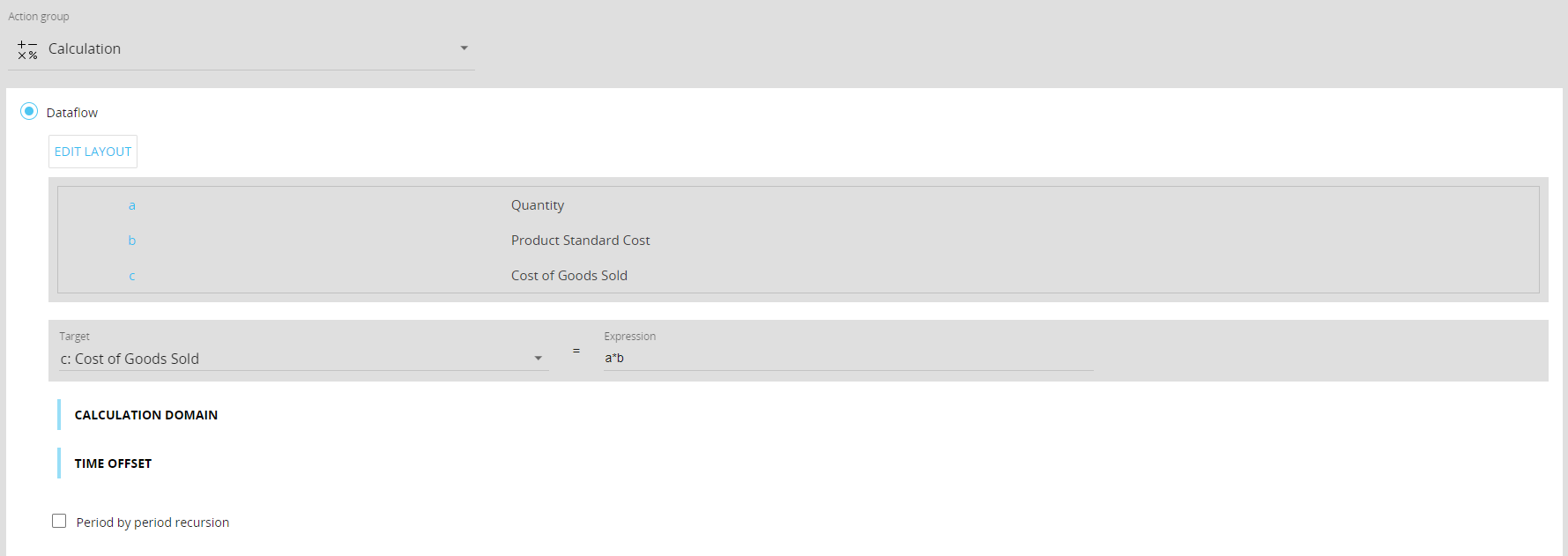Before executing the calculations, Board checks the structural congruence of the Cubes involved: both the Cubes "Quantity" and "Cost of Goods Sold" are dimensioned by Month, but the Cube "Product Standard Cost" is based on the Time Entity "Year". In this case, Board automatically uses the "Month-Year" relationship to perform the calculation between Cubes with a different structure.

What the Dataflow will execute can be described as follows: ”For each combination of Month, Product, and Customer, multiply the Quantity by the Product Standard Cost of the Product considered in the Year to which the Month belongs”.

The automatic use of relationships is not limited to Time Entities, but applies to any hierarchical relationship defined in the Data model.

## Calculation domain

The Calculation Domain allows to explicitly define the range of Cube cells that will be processed, thus simplifying the definition of Dataflow regardless of the structure of the Cubes and, at the same time, optimizing performance by considering only the desired portion of the Cubes.

The Dataflow calculations are performed by default only on the non-null cells of the associated Layout. The Dataflow will apply the formula to each combination of the members of Entities that are in the Structure of the target Cube: not to every potential combination, though, but only to those which have a non-zero value on at least one of the Blocks of the Layout.

Each of those combinations is called a tuple. Through the Calculation Domain settings you can extend or reduce the range of tuples processed.

The domain can be limited or expanded using the following options:

• Limit calculation to tuples where values of at least one cube of the Layout are non-zero (default). The domain is limited to the tuples that are non-zero in at least one of Block of the associated Layout ignoring the density/sparsity of the target Cube and considering only the Cubes that can be aggregated to the target Cube structure on the Entities that are not extended
• Execute calculation on existing sparse combination of target cube for all the dense (back-compatible). The Dataflow in Board 10.x wrote data only on existing combinations in sparse structures and for each dense combination of Entity members included in the target Cube structure and this option will automatically set the domain to what it was in version 10.x of Board: this is particularly useful in case of upgrades from version 10.x, since Dataflows will be using the same behavior of cell-based Dataflows in versions 10.x.

If used with HBMP Dataflows, this option can have a negative impact on performance.

• Limit calculation to tuples of the Cube. The domain is limited to the tuples that are non-zero in a specific Cube of the Data model, selected in the "Cube" dropdown menu. This option limits the domain so that the Dataflow writes data only on existing combinations of a given Cube

If the Cube configured in the dropdown menu is not found, the default option will be used.

### Extend options

"Limit" options can be combined with the extend options, so the Dataflow calculation will be performed on existing combinations in the Cube set in the limit option and for each combination of extend Entities in a Select.

The "Extend calculation on new tuples for all members of" option expands the calculation domain by evaluating also the combinations that are zero in the associated Layout for all members of a given set of Entities of the target Cube.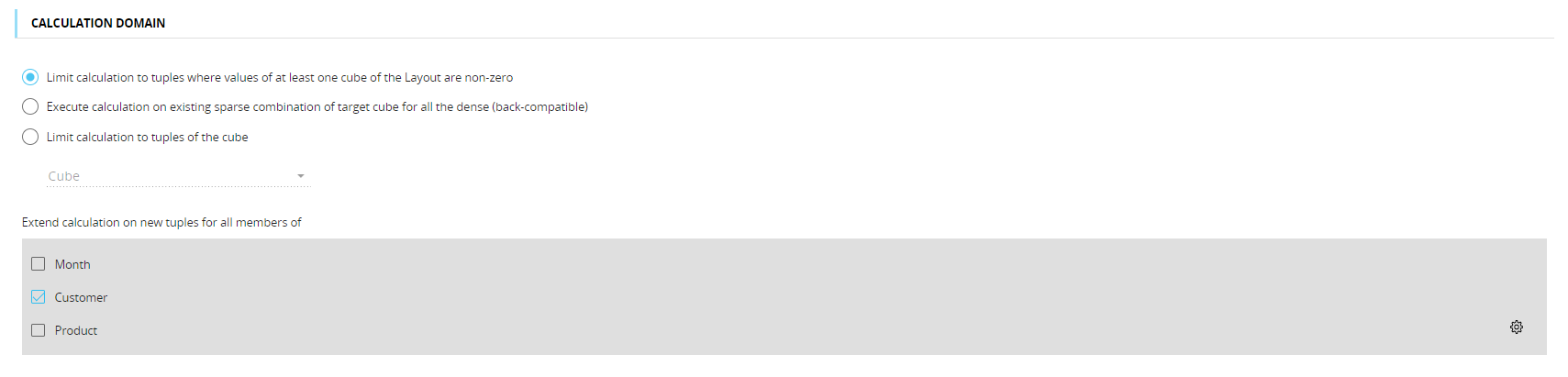If the Dataflow Layout includes an Entity as a Data Block, all Entities in the "Extend calculation on new tuples for all members of" option will be automatically considered in the definition of the calculation domain.

The maximum number of tuples included in the calculation domain by the "Extend calculation on new tuples for all members of" option cannot exceed 2^31. To find that number based on your settings, calculate the product of the members in the active selection that belong to the Entities on which the extend was set.

## Time offset

The Time Offset is a feature of the Dataflow that allows you to shift Cube values on the time dimension. The shift can be absolute, based on a specific date (Absolute Offset option), or relative (Relative Offset option), shifting values forward or backward for a given number of time periods defined by the Cube set in the "Target dynamic offset" field.

The Relative offset option shifts Cube values forward or backward in time based on the number of periods specified in the Cube set in the "Target dynamic offset" field. The time shift can be forward (Forth option) or backward (Back option).

For the Relative offset option to work as expected, you must select a Cube containing integer values in the "Target dynamic offset" field.

The Absolute offset shifts values to the date specified in the Cube selected in the "Target dynamic offset" field.

For the Absolute offset option to work as expected, you must select a Cube containing dates (a Date Cube) in the "Target dynamic offset" field.

The Time Offset feature is located in the "Time Offset" dropdown menu.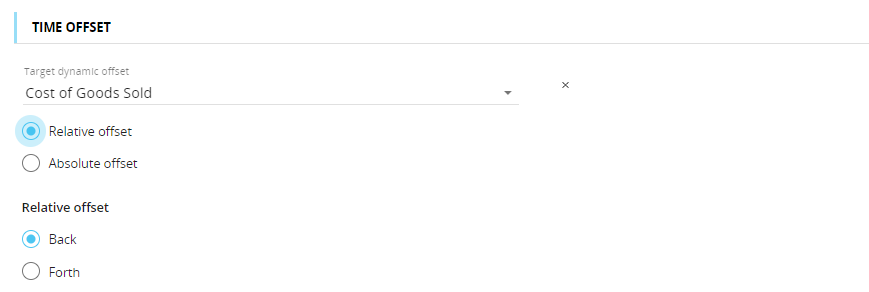The Dataflow step always runs in replace mode, except when the time offset options are in use. This should be considered, for example, when the target Cube is also a factor Cube and by applying a time offset to it the slice of the target Cube that gets cleared before writing is shifted accordingly along the chosen time dimension.

If, after the time offset application, two or more values fall in the same tuple, they are summed. For example, the January value with offset 2 and the February value with offset 1 would both fall in the March tuple: in this case, those values are summed and the formula result is then written in the March tuple.

### Period by Period recursion

If the target Cube of a Dataflow is also a source for that same Dataflow and the source Block is set to period offset negative, the “Period by Period recursion” option can be enabled.

This option can only be enabled if all the previously mentioned requirements are met.

The “Period by Period recursion” checkbox allows the Dataflow to use the results of the first period's calculation as a source for the next period's calculation.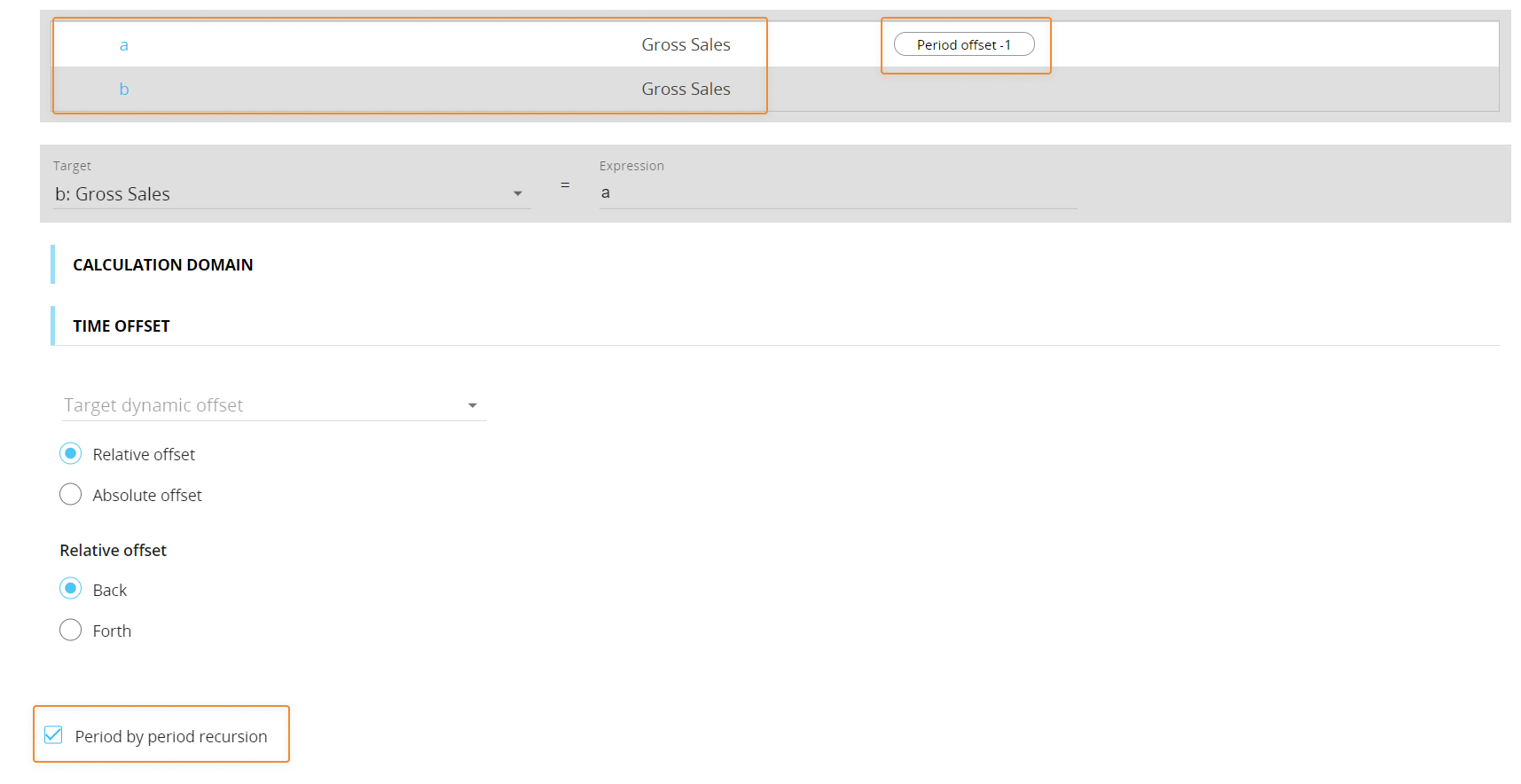This option is particularly useful for those calculations where the result of the Dataflow for the first period needs to be used as the source for the following one.

The “Period by Period recursion” setting is also referenced in the Impact Analysis section of the Data model affected by the Dataflow calculations:

• Recursion Active. The option is active
• Recursion Available. The option can be selected (but it might be inactive)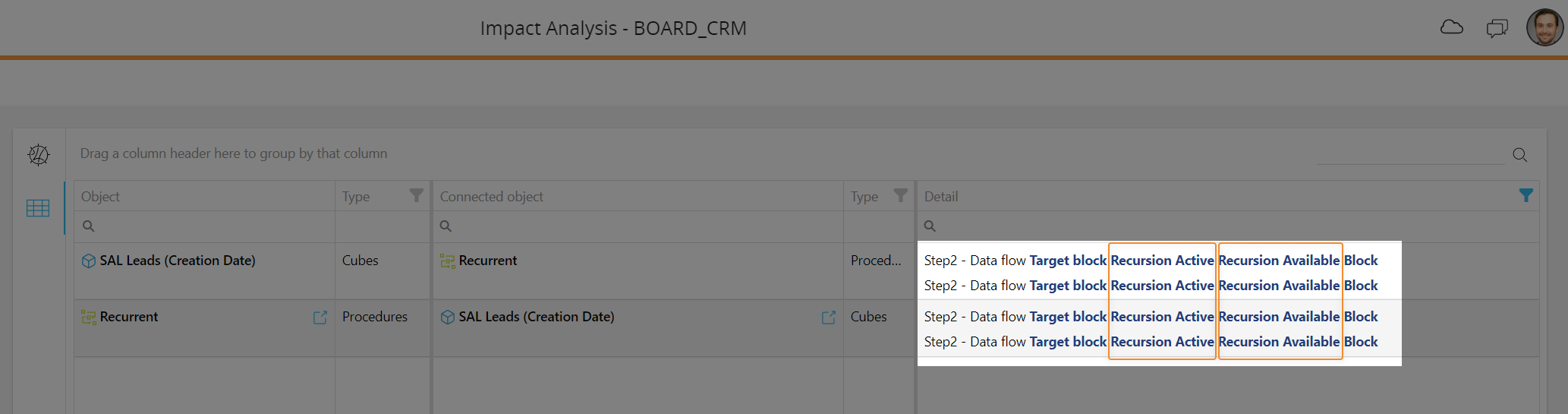## Dataflow rules and guidelines

• Evaluation of the formula is always done at the aggregation level of the target Cube. If one or more Cubes referenced in the formula (factor Cubes) have different structures from the target Cube, they will be brought to a congruent level before the formula is evaluated, according to the following rules:
• When a Cube referenced in the formula (the factor Cube) has one dimension that the target Cube does not have, this dimension will be aggregated. For example, if a Cube in the formula is structured by Customer/Product/Month and the target Cube only by Product/Month, then values in the factor Cube are aggregated for all selected Customers before executing the calculation.
The structure of the Cubes mentioned above would be the following:  Entities in the Cube structure Factor Cube Target Cube Month X X Product X X Customer X

• When the target Cube has a dimension that a factor Cube does not have, the values in the factor Cube are repeated for each member of the exceeding dimension of the target Cube. This process is called "allocation" and considers only those tuples in the calculation domain. For example, if one of the factor Cubes is Commission Percentage, structured by Salesperson/Month, and the target Cube is Commission Percentage, structured by Salesperson/Product/Month, the same Commission Percentage value will be repeated for each Salesperson/Month for all products.
The structure of the Cubes mentioned above would be the following:  Entities in the Cube structure Cube "Commission Percentage" Cube "Commission Percentage" (target) Month X X Product X Salesperson X X

• When a factor Cube is structured by a less aggregate Entity than the target, the factor is aggregated to the same level of the target. For example, if one of the factor Cubes is structured by Product/Month, and the Target is structured by Product/Quarter, then the factor values by Month will be summed to get to values by Quarter through to the Month-Quarter relationship.
The structure of the Cubes mentioned above would be the following:  Entities in the Cube structure Factor Cube Target Cube Month X Quarter X Product X X

• If a factor Cube is structured by a more aggregate Entity than the target Cube, the value of the parent occurrence is determined through the parent-child relationship only for those tuples in the calculation domain. For example, if one factor Cube is "%Royalty" structured by Product Brand/Year and the target Cube is "Royalty Value" structured by Product/Month, and a Product→Product Brand relationship is in place, then the "%Royalty" value for each Brand will be applied to each Product based on its associated Brand.
The structure of the Cubes mentioned above would be the following:  Entities in the Cube structure Cube "%Royalty" Cube "Royalty Value" Month X Year X Product X Product Brand X

• The Dataflow step, just like the most part of other steps, adheres to the active Selection. This should be considered, for example, when you need to copy only a portion of a Cube and not the whole Cube.

The active Selection influences only the Cubes that have Entities on which the selection is made as dimensions.

• In the Layout editor, available Time functions, Analytical functions, Block references (Refer to), and the Total by function should only be applied to source Cubes. This should be considered, for example, when you need to copy a portion of a Cube into a different position on the target Cube: in this case, the select must always define where to copy data on the target Cube.

## Dataflow Performance

The execution time of a single Dataflow step depends on different factors: the Dataflow configuration, the structure of the Cubes involved, their size, and the selections applied to the dimensions of Cubes.

The most accurate way of evaluating the Dataflow speed is through the analysis of Logs related to the Data model where the Procedure containing the Dataflows has been executed: log details include, which algorithm type has been used for the Dataflow calculations and the elapsed time, among other information.

### Types of algorithms

The Dataflow step may use different types of algorithms for its calculations, depending on certain conditions related to the structures of the Cubes involved and the formula. They are the following:

• Straight. This calculation algorithm is only used to copy Cubes (or portions of them, depending on the currently active Select) with formulas such as b=a and when the calculation domain options are in the default configuration
• Tuples [intersect]. This calculation algorithm is only used when the entered formula is a multiplication or a division and only factors that are non-zero are considered
• HBMP. This calculation algorithm is used if the following conditions are met:
• The target Cube is the last Block configured in the Layout and it cannot be Block "a" (for example, a c=b*a Dataflow can use the HBMP+ algorithm, while a a=b*c Dataflow will not)
• Cube structures are as similar as possible and no allocations occur. If the target Cube structure is a subset of one of the source Cube structures, the Dataflow will use the HBMP algorithm
• The expression c=a*b may use the HBMP algorithm, depending on the structures of the Cubes involved. In other words, only expressions that contain the * and / operators (multiplications or divisions) can use the HBMP+ algorithm
• No Rules and the "Refer to" and "Total by" functions are used in the Layout
• The Layout contains only Cubes as Blocks
• JOIN. This calculation algorithm is only used when the calculation domain options are in the default configuration and the Layout contains three Cubes whose structures meet the following conditions:
• One factor Cube has n dimensions in its Structure, where is any number
• The Structure of the second factor Cube has:
• the same extra dimension as the target Cube with respect to the first factor Cube
• at least a dimension of the first factor Cube
• The target Cube has in its Structure all the dimensions of the factor Cubes and exactly one more than the first factor Cube (n+1)
• CONSTANT. This calculation algorithm is only used when the Layout contains just the target Cube, with formulas such as a=constant (for example a=2, a=4, etc.).

You can view the algorithm type used by the step in the Logs section of the Data model, in the "D.Flow Mode" column. In that column, the algorithm types may be followed by a "+" sign: this means that no spreadsheet components have been instantiated for evaluating the formula, resulting in a slightly faster calculation time. In other words, this happens when the expression is a basic mathematical operation (i.e. an addition, subtraction, multiplication, or division) and is evaluated directly by Board.